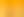Real World, Real Numbers: Ratio and Proportions

# Stages1. Building Understanding of Ratios

EXPLORE

Suggested Learning Intentions

• To recognise the relationships between quantities or measures in ratios

Sample Success Criteria

• I can model a ratio using diagrams, objects and other manipulatives
• I can describe ratios as a comparison between quantities
• I can increase or decrease quantities while keeping the ratio constant
• I can identify equivalent ratios
EXPLORE

2. Finding the Unknown in Ratios

EXPLORE

Suggested Learning Intentions

• To build fluency in applying our understanding of ratios to solve problems

Sample Success Criteria

• I can calculate the quantity for each part of a ratio
• I can calculate the unknown value of a ratio
• I can demonstrate my thinking and justify my solutions using a range of manipulatives
EXPLORE

3. Comparing Ratios

EXPLORE

Suggested Learning Intentions

• To apply our knowledge of equivalence to compare ratios

Sample Success Criteria

• I can simplify and compare ratios
• I can identify if two ratios have the same value
• I can describe the effects of adding parts of a ratio
• I can model and demonstrate my thinking using a range of manipulatives
EXPLORE

4. Finding the Better Deal

EXPLORE

Suggested Learning Intentions

• To evaluate different rates and prove that one is better than the other

Sample Success Criteria

• I can identify ratios as quotients and rates
• I can find the best deal
• I can compare rates
• I can use a range of manipulatives to model and justify my thinking
EXPLORE